# Common Mistakes when Solving Trig Equations

## Brian McLogan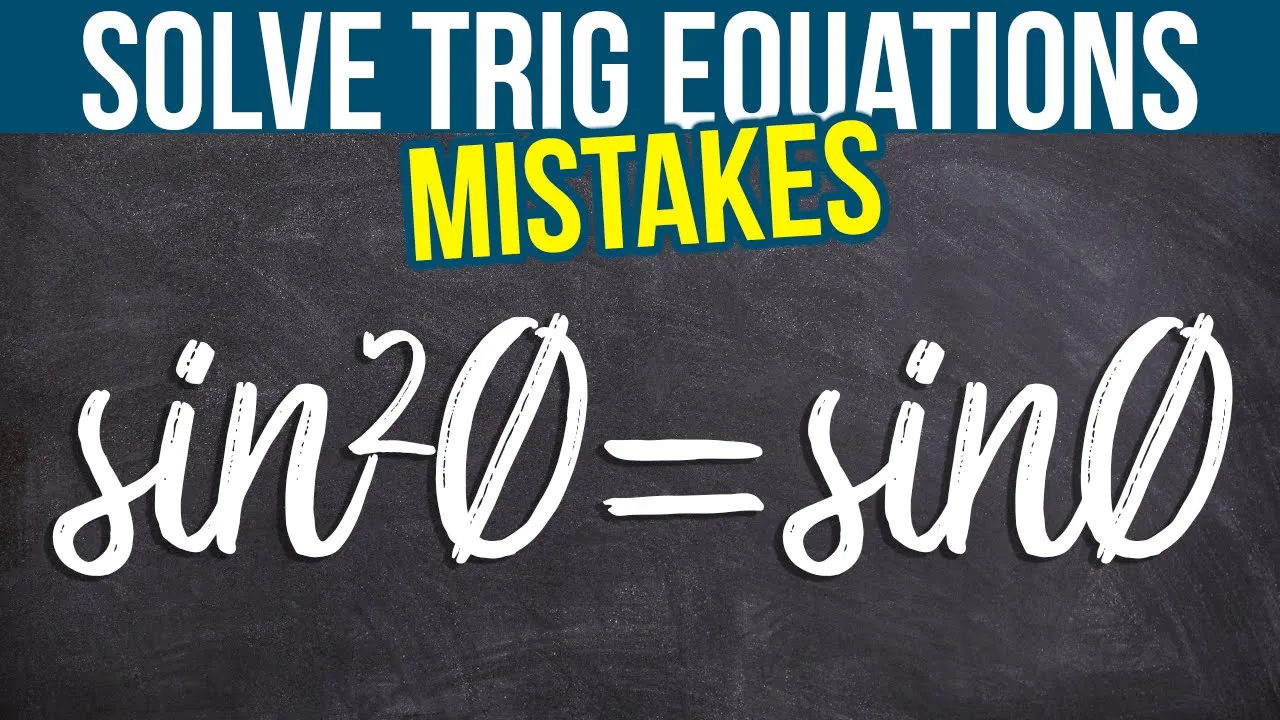Sin²θ = sinθ is a problem that I see students make mistakes on time and time again. I want to show you some mistakes that I see students often make so you don’t make the same ones. We’ll also look at this problem and how to approach it so you can solve it correctly. I’ll find all of the solutions and the solutions between 0 and 2π.

## Mistake #1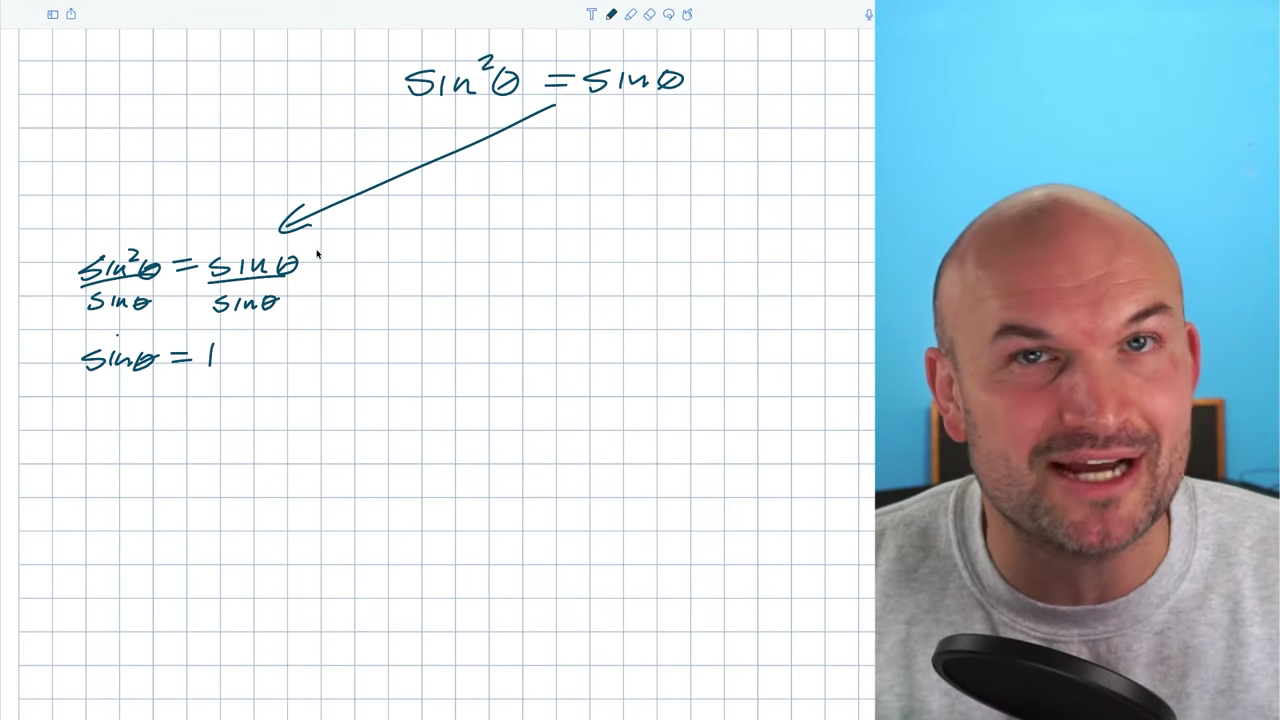With a sinθ on both sides, it’s often tempting for students to just divide by sinθ and eliminate the one on the right. The problem is, you’ve now gone from what was a quadratic equation with two solutions to a linear equation with only one. Dividing by sine is technically a solution to this equation, but by dividing by sinθ and reducing the power of the equation, we’ve left off the other solution. Please be careful when dividing by trigonometric functions not to reduce the power of the equation.

## Mistake #2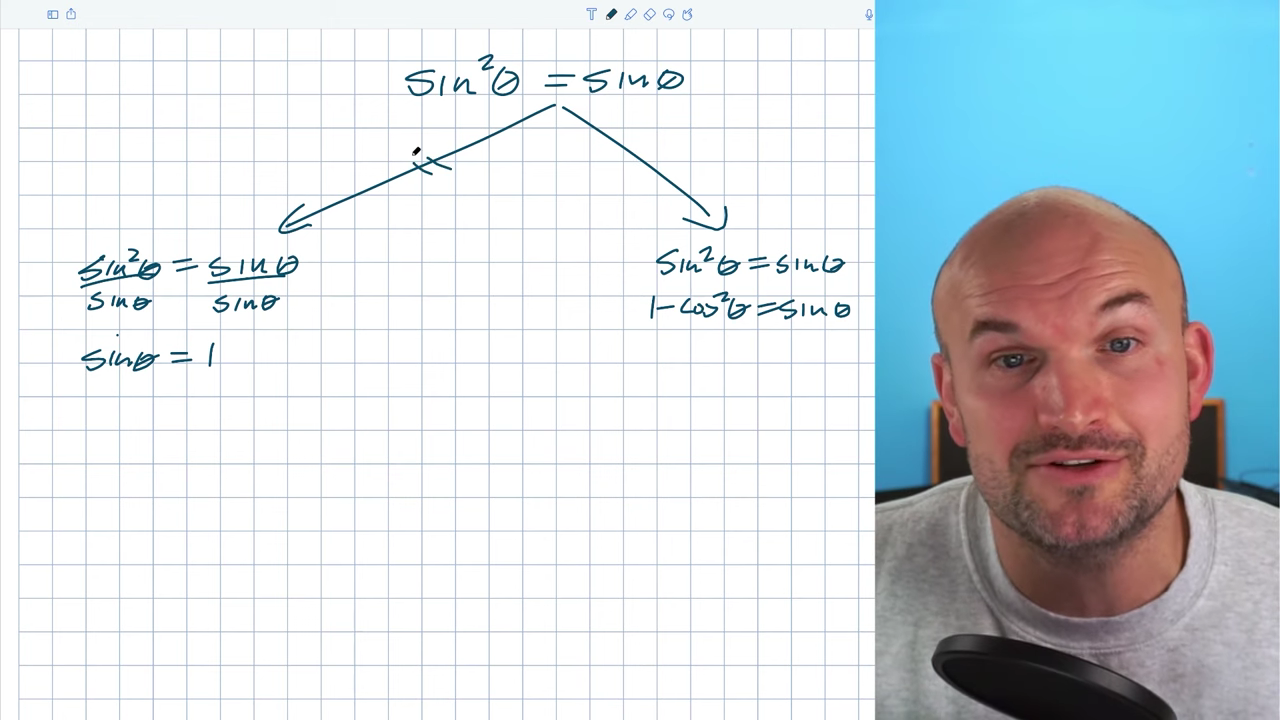I often tell my students to think of Pythagorean identities whenever they see something squared. Immediately, they want to convert sin² into cosines. There’s nothing wrong with this, but now we’re trying to solve an equation with two different trigonometric functions, which is not ideal. It’s preferable to solve using the same trigonometric function, in this case, sines.

## Solving the Problem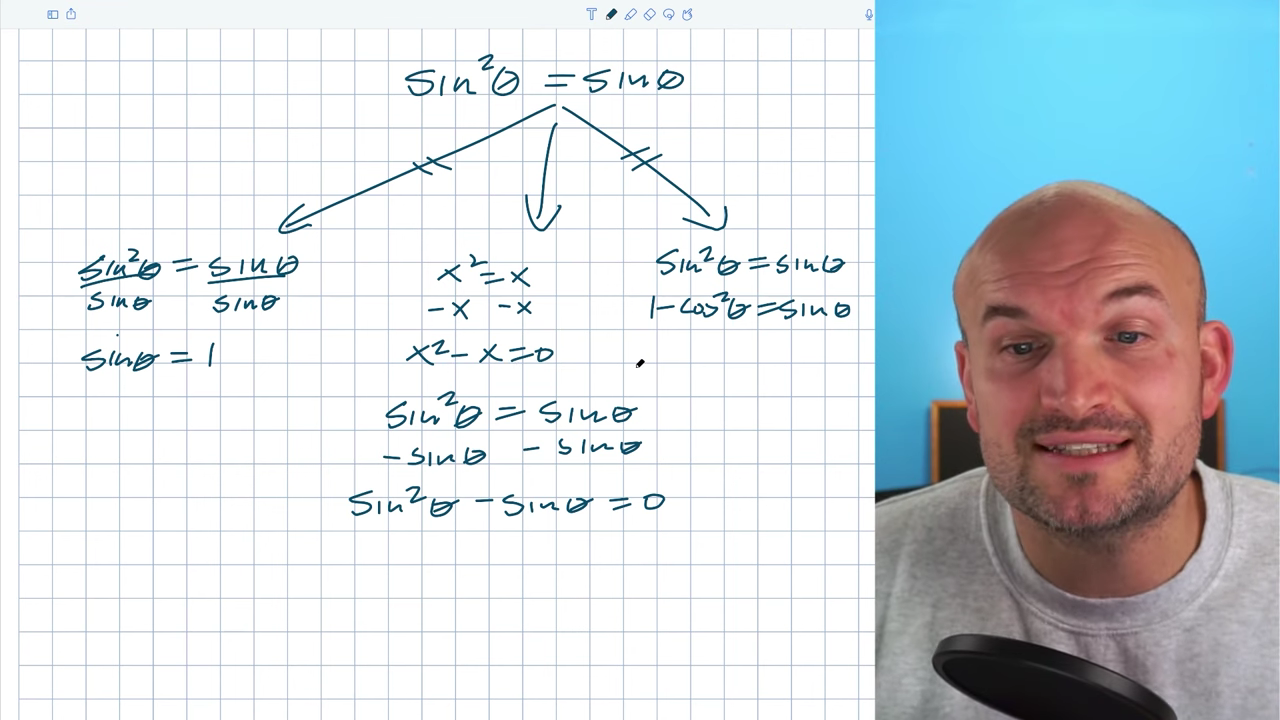So, what do we do? I want to go back through and think about this in terms of like variables again. You wouldn’t divide X² = X by X. With quadratic equations, we want to get all the variables to the same side: X² – X = 0. Now, I can use factoring to rewrite this as a product. When you have a product, you can use the zero product property.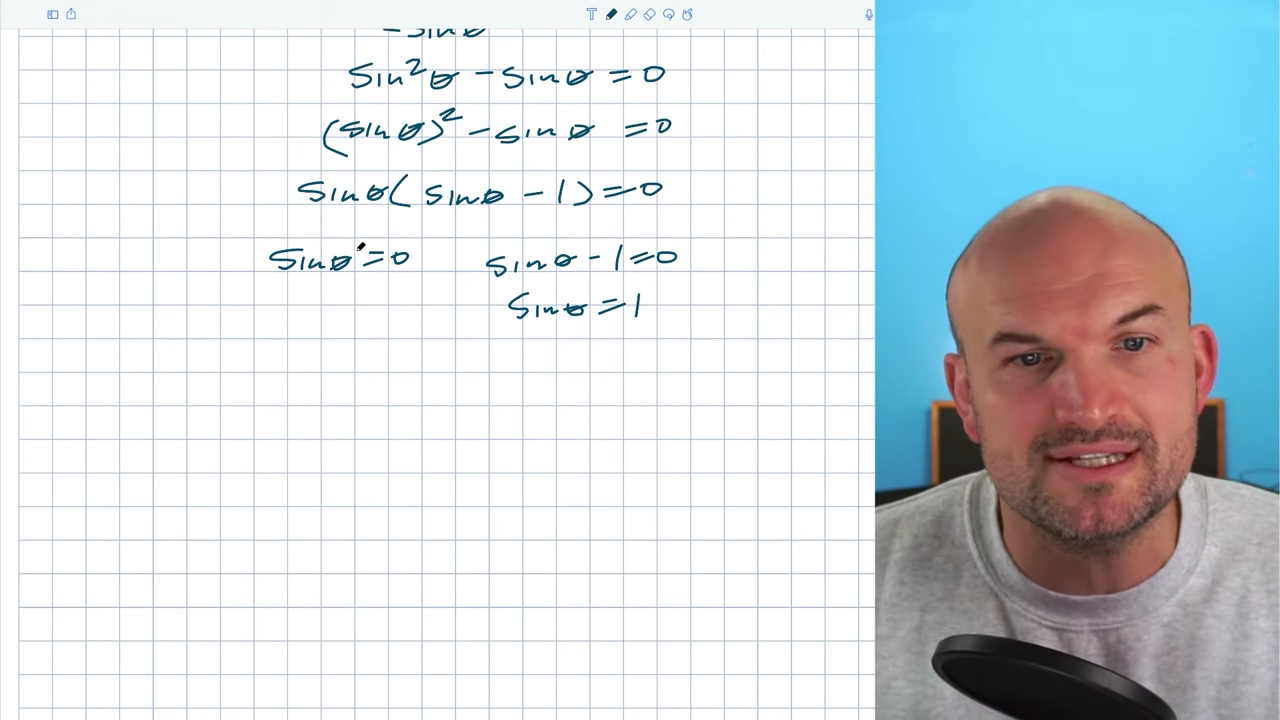Now we have Sin²θ – sinθ. We cannot subtract these because they’re not like terms. Sin²θ is quadratic, and sinθ is linear. However, we can recognize that they both have a sinθ in common, just like both parts have X in common in the other equation. We can factor out the X to be left with X(X-1) = 0. Sin²θ can be rewritten as (sinθ)² – sinθ = 0. Because it’s a common sign, I can now factor out the sinθ and be left with sinθ(sinθ – 1) = 0. When your product is zero, you can set each factor equal to zero: sinθ = 0 and sinθ – 1 = 0. This goes back to the original solution of sinθ = 1. Mistake #1 gave us the solution but left off sinθ = 0, so it’s important to watch out for.

## Solutions Using Unit Circle

To solve these equations, we must understand the angle that will produce these results. The equations ask, the sine of what angle = 0 and the sine of what angle = 1? The easiest way to understand these angles is to look at the y coordinates using the unit circle. 0 and 1 are coordinate values on our unit circle, so if I draw a unit circle, I can recognize the y coordinate as 1 at 0,1 and the y coordinate as zero at two other angles.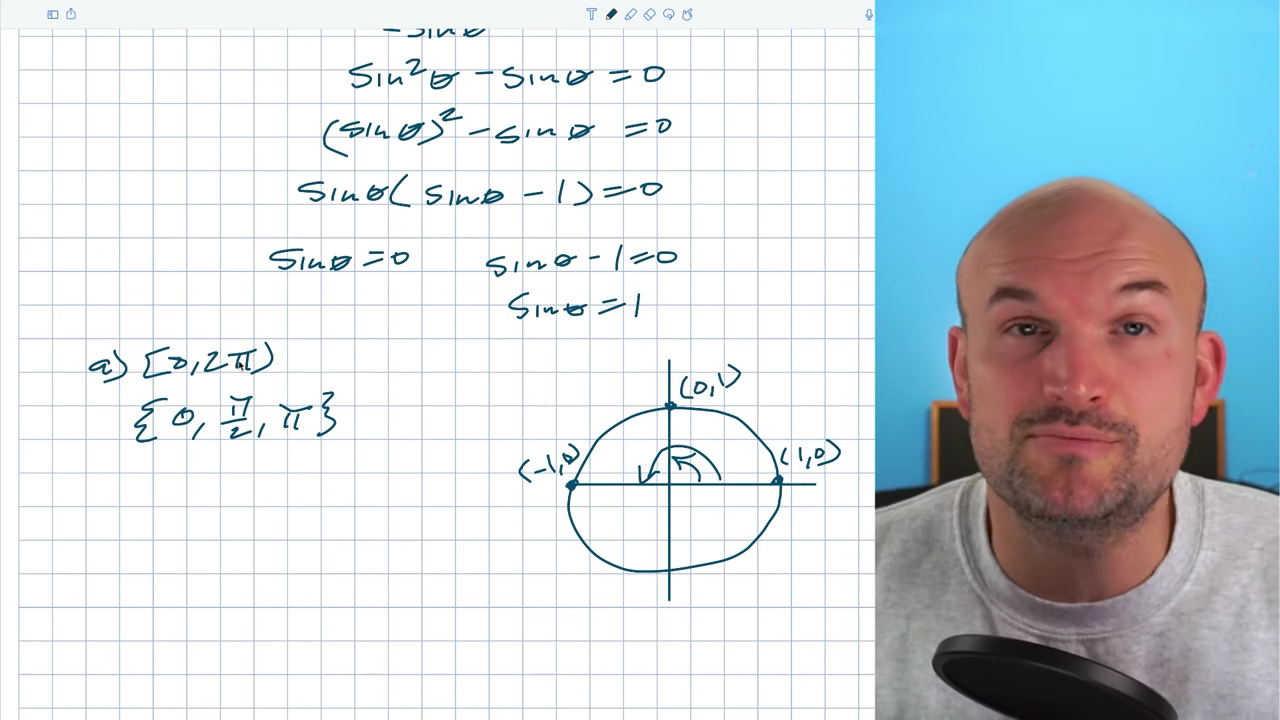Now, I need to figure out what these angles are to find the solutions between 0 and 2π. When you’re trying to find the solutions on the interval of 0,2π, you’re finding the solutions on the unit circle. For the interval 0,2π, 0 will usually be included, and 2π will not. In these solutions, we have the first point at 0 radians. The next solution is π. All the way around would be 2π, so halfway around the circle is π. This angle is half of that, which is π/2. 0,2π, 0, π/2, and π are the solutions on 0,2π.

## All Solutions

What if we want to find all solutions from -∞ to ∞? There are no restrictions. I can keep finding the angle where sinθ is the n = 0 and sinθ = 1. The sine graph will continue its pattern indefinitely. Just add 2π to each of these angles because adding 2π takes me back to the same angle. The sine of that angle, which in this case would be 4π, is equal to zero.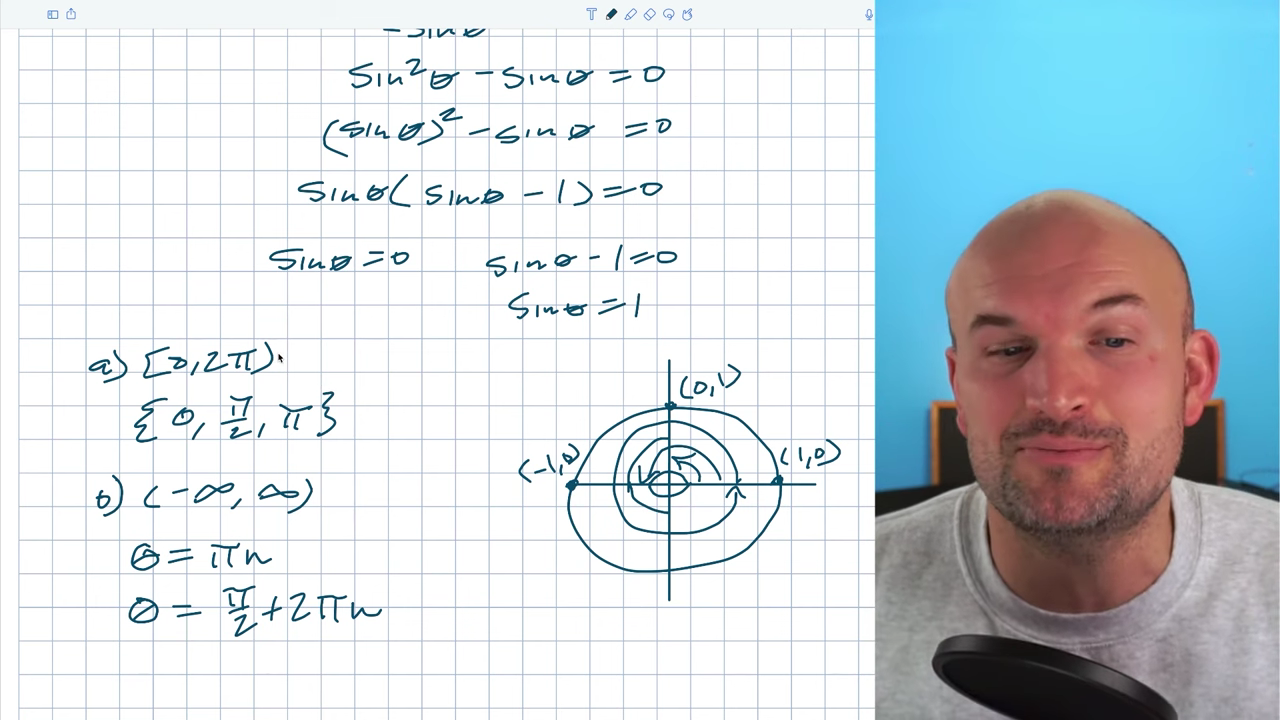You can also go in the negative direction and subtract 2π. Doing the sine of -2π in the negative direction, I still get a zero. To write all of the intervals of 0 and 2π, I could just write 0 + 2πn, where n represents a positive or a negative number. Writing 2πn is not the most efficient way to write all solutions. The distance between 0 and π is π. If I keep adding or subtracting π, I’ll keep bouncing between these two solutions. Writing θ + πn will satisfy both solutions with one equation because n represents any integer.

For my second solution of π/2, there’s no interval that I can keep adding. Adding π/2 would take me to π, and adding π/2 again would take me to 3π/2, which is not a solution. I have no choice but to keep adding 2πn.

That’s how you find the solutions on 0,2π or all the solutions. It’s very important to recognize when you need to factor because we don’t want to lose a solution or get stuck with identities. I hope this was helpful. If you want more examples or resources, check out the links below. Cheers!

More Examples Solve Trigonometric Equations: https://www.youtube.com/playlist?list…